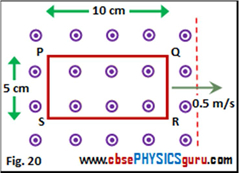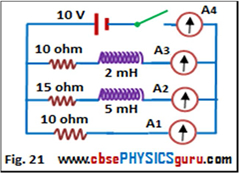View Solutions

1. A square wire loop of 4 cm sides is perpendicular to a magnetic field of 5 mT. Calculate the magnetic flux through the loop.

2. A rectangular coil of area 2 cm2, having 2000 turns is placed perpendicular to a magnetic field of flux density 0.02 Wb/m2. If the field drops to zero in 0.01 sec, what is the induced e.m.f.?

3. The vertical component of the earth's magnetic field in a place is 3 x 10-5 T. What is the potential difference between the ends of the axle of a car which are1.5 m apart, when car's velocity is 20 m/s?

4. A fan blade with a length L rotates with a frequency f perpendicular to a magnetic field B. Find the potential difference between the centre and end of the blade.

5. A circular coil of 100 turns and an area of 10 cm2 rotates about a diameter with an angular velocity 100 rad/s in a uniform magnetic field of 0.10 T. The axis of rotation is perpendicular to the field. The coil has a resistance of 10Ω. The emf induced in the coil is supplied to an external resistance of 10 Q. Calculate the peak current flowing through the external resistance.

6. A rectangular coil of length 20 cm and width 15 cm having 50 turns rotates in a field of 20 T at the rate of 900 rpm. What is the value of the induced voltage when the plane of the coil makes an angle of 600 with the field?

7. Calculate the self-inductance of a solenoid having 1000 turns and length 1 m. The area of crosssection is 10 cm2 and relative permeability μr = 1000.

8. Calculate the emf induced in a 4 H inductor in which the current change from 10 A to 5 A in 10 ms.

9. The winding of an electromagnet used in hoisting machine has 1000 turns and a resistance of 20 ohm. The magnetic flux produced in the winding at the exciting voltage of 200 volt is 0.01 Wb. Calculate the energy stored in the magnetic field.

10. The co-efficient of mutual inductance of two coils is 2 H. A current of 2 A passing through the first coil is switched off in 1/2000 s. Calculate the emf induced in the second coil.

11. A solenoid of 100 turns and radius 0.02 m is placed inside a long solenoid of radius 0.2 m and having 5000 turns per meter such that the axes of two solenoids coincide. The current in the outer solenoid is decreased at a constant rate from + 3A to — 1A in 0.02 sec. Find the emf induced in the inner coil.

12. A rectangular wire loop of sides 8 cm and 2 cm with a small cut is moving out of a region of uniform magnetic field of magnitude 0.3 T directed normal to the loop. What is the emf developed across the cut if the velocity of the loop is 1 cm/s in a direction normal to the (a) longer side, (b) shorter side of the loop? For how long does the induced voltage last in each case?

13. A circular coil of radius 8.0 cm and 20 turns is rotated about its vertical diameter with an angular speed of 50 rad/s in a uniform horizontal magnetic field of magnitude 3.0 × 10-2 T. Obtain the maximum and average emf induced in the coil. If the coil forms a closed loop of resistance 10 O, calculate the maximum value of current in the coil. Calculate the average power loss due to Joule heating. Where does this power come from?

14. A pair of adjacent coils has a mutual inductance of 1.5 H. If the current in one coil changes from 0 to 20 A in 0.5 s, what is the change of flux linkage with the other coil?

15. A jet plane is travelling towards west at a speed of 1800 km/h. What is the voltage difference developed between the ends of the wing having a span of 25 m, if the Earth's magnetic field at the location has a magnitude of 5 × 10 - 4 T and the dip angle is 30°.

16. An air-cored solenoid with length 30 cm, area of cross-section 25 cm2 and number of turns 500, carries a current of 2.5 A. The current is suddenly switched off in a brief time of 10- 3 s. How much is the average back emf induced across the ends of the open switch in the circuit? Ignore the variation in magnetic field near the ends of the solenoid.

17. A closed coil consists of 500 turns on a rectangular frame of area 4 cm2 and has a resistance of 50 ohm. The coil is kept with its plane perpendicular to a uniform magnetic field of 0.2 T. Calculate the amount of charge flowing through the coil if it is rotated through 1800.

18. The plane of a loop of wire is at right angles to a uniform magnetic field of magnitude 4.0 x 10 - 2 T. The loop is moving across the field at a constant speed. At the instant shown in the figure 20, the loop is completely within the field. To the right of the dotted line, the field is zero. The resistance of the loop is 2.0 Ohm.
1. Which two sides have an induced EMF across them?
2. Why is no current driven by the two induced EMF's?
3. At the instant of the diagram, which side of the loop: (i) has a positive charge? (ii) has a negative charge?
4. Describe the position of the loop when a current first exists.
5.6. Describe the change in the magnetic flux through the loop while it is leaving the field.
7. A magnetic field surrounds the loop when a current exists. Describe the direction of this field on the inside the loop.
8. Is the direction of the current in the loop clockwise or anticlockwise?
9. The current is driven by the induced EMF across PS. Calculate the magnitude of this EMF.
10. Calculate the value of the current.
11. Determine the magnitude and direction of the induced magnetic force acting on side PS while the loop is leaving the field.
12. Determine the magnitude and direction of the external force acting on the loop.
13. What is the magnitude of the magnetic flux through the loop at the instant shown in the figure?
14. What is the time interval between sides QR and PS crossing the dotted line?
15. Calculate the magnitude of the induced EMF using the formula ε = ΔφB / φt.
16. Describe the position of the loop when the current first becomes zero.
17. Calculate the total charge transferred past a point in the loop while the current was present.
18. What will be the current if the loop moved at 0.25 m s-1?
19. What will be the total charge transferred if the loop were moving at 0.25 m s-1?

19.20. Find the reading of each ammeter in the circuit diagram shown in the figure 21:
1. just after switch is closed
2. long after switch is closed.

21. The figure 18 shows the coil of an ac generator every quarter turn during one rotation. In the first diagram, the coil's plane is perpendicular to the magnetic field. At t = 0 the coil is rotated at a constant rate in clockwise direction about its axis.
1. What is the magnetic flux through the coil at t = T/4?
2. Describe the change in magnetic flux through the coil during the first quarter turn.
3. Starting from A, describe the direction of the induced current in the first quarter turn e.g. ABCDA.
4. Describe the change in magnetic flux through the coil during the second quarter turn.
5. Describe the direction of the induced current in the second quarter turn.
6. Describe the current in words during one complete turn of the coil.
7. Describe the coil's orientation relative to the field when the current reverses direction.
8. Describe the orientation of the coil relative to the field when the current is a maximum.

CBSE Electromagnetic Induction ( With Hint / Solution)
Class XII (By Mr. Ashis Kumar Satapathy)
email - [email protected]